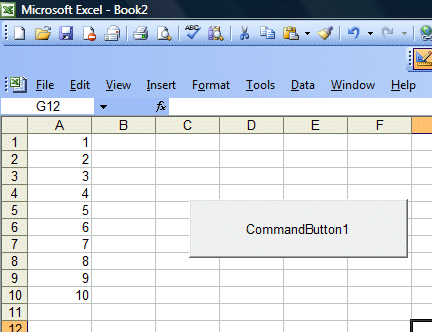Lesson 14: The For...Next Loop

In lesson 12, we have learned how to handle decisions making process using If...Then...Else struture. Another procedure that involves decisions making is looping. Looping is a procedure that repeats many times until a condition is met. There are two kinds of loops in Excel VBA, the For.......Next  loop and the Do...Loop . In this lesson, we shall deal with with the For...Next Loop and we shall learn the Do...Loop in the next lesson.

The For....Next Loop event procedure is written as follows:

For counter=startNumber to endNumber (Step increment)

One or more statements

Next

To demonstrate the For....Next loop in Excel VBA, here are two examples:

Example 1:

Private Sub CommandButton1_Click()
Dim i As Integer
For i = 1 To 10
Cells(i, 1).Value = i
Next
End Sub

In this Excel VBA program, you place the command button 1 on the spreadsheet then click on it to go into the Visual Basic editor. When you click on the button , the Excel VBA program will fill cells(1,1) with the value of 1, cells(2,1) with the value of 2, cells(3,1) with the value of 3......until cells (10,1) with the value of 10. The position of each cell in the Excel spreadsheet is referenced with cells(i,j), where i represents row and j represent column.

In Example 2,we use the nested loop to put the values of i+j from cells(1,1),cells(1,2),cells(1,3),cells(1,4),cells(1,5) ..........until cells(10,5). The code and output are shown below.

¡¡

Output of Example 1Example 2

Private Sub CommandButton1_Click()
Dim i, j As Integer
For i = 1 To 10
For j = 1 To 5
Cells(i, j).Value = i + j
Next j
Next i
End Sub
¡¡